# Support Vector Machine Algorithm - Machine Learning

Rating: 5

6918

Support Vector Machines (SVMs, also support vector networks are supervised learning models with associated learning algorithms that analyze data used for classification and regression analysis. It is formally defined by a separating hyperplane. In other words, given labelled training data (supervised learning), the algorithm outputs an optimal hyperplane which categorizes new examples.

Learn how to use Machine Learning, from beginner basics to advanced techniques. Enrol for Free Machine Learning Training Demo!

If we wish to categorize new unseen objects into two separate groups based on their properties and a set of known examples, which are already categorized. A good example of such a system is classifying a set of new documents into positive or negative sentiment groups, based on other documents which have already been classified as positive or negative. Similarly, we could classify new emails into a spam or non-spam, based on a large corpus of documents that have already been marked as spam or non-spam by humans. SVMs are highly applicable to such situations.

A Support Vector Machine models the situation by creating a feature space, which is a finite-dimensional vector space, each dimension of which represents a "feature" of a particular object. In the context of spam or document classification, each "feature" is the prevalence or importance of a particular word.

## Hyperplane

the hyperplane is a line that linearly separates and classifies a set of data.

### Support Vector

Support vectors are the data points nearest to the hyperplane, the points of a data set that, if removed, would alter the position of the dividing hyperplane.

### Margin

The distance between the hyperplane and the nearest data point from either set is known as the margin.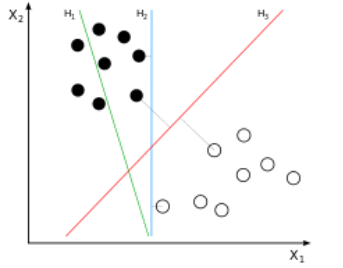H1 does not separate the classes. H2 does, but only with a small margin. H3 separates them with the maximum margin.

Related Article: Machine Learning Tutorial

### Identify the Right Hyperplane

Identify the right hyper-plane (Scenario-1): Here, we have three hyper-planes (A, B and C). Now, identify the right hyper-plane to classify star and circle.

We need to remember a thumb rule to identify the right hyper-plane: “Select the hyper-plane which segregates the two classes better”. In this scenario, hyper-plane “B” has excellently performed this job.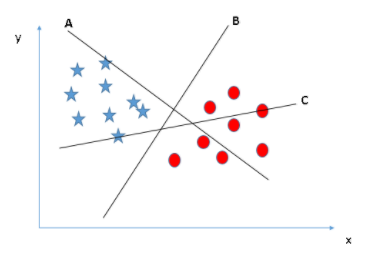Identify the right hyper-plane (Scenario-2): Here, we have three hyper-planes (A, B and C) and all are segregating the classes well. Now, how can we identify the right hyper-plane?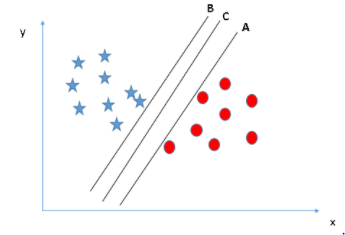Here, maximizing the distances between nearest data point (either class) and hyper-plane will help us to decide the right hyper-plane. This distance is called as Margin. Let’s look at the below snapshot: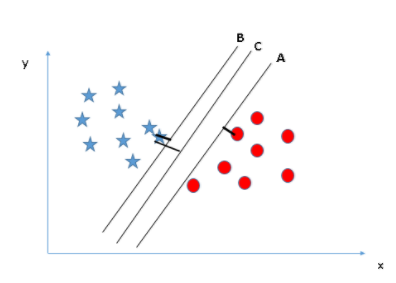Above, you can see that the margin for hyper-plane C is high as compared to both A and B. Hence, we name the right hyper-plane as C. Another lightning reason for selecting the hyper-plane with higher margin is robustness. If we select a hyper-plane having low margin, then there is high chance of miss-classification.

The perfect guide for getting started to applied machine learning. Access to free..Machine Learning Tutorials

Identify the right hyper-plane (Scenario-3):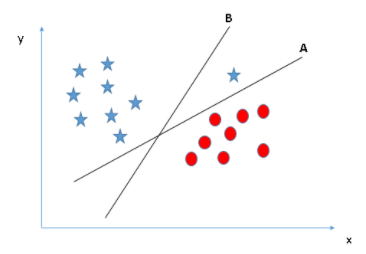We may have selected the hyper-plane B as it has a higher margin compared to A. But, here is the catch, SVM selects the hyper-plane which classifies the classes accurately prior to maximizing margin. Here, hyper-plane B has a classification error and A has classified all correctly. Therefore, the right hyper-plane is A.

Can we classify two classes (Scenario-4)?: Below, we are unable to segregate the two classes using a straight line, as one of star lies in the territory of other(circle) class as an outlier.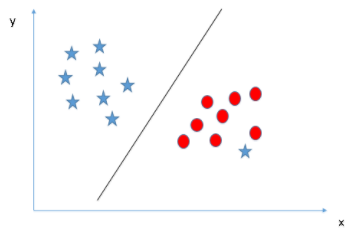As I have already mentioned, one star at other end is like an outlier for star class. SVM has a feature to ignore outliers and find the hyper-plane that has a maximum margin. Hence, we can say, SVM is robust to outliers.

Find the hyper-plane to segregate to classes (Scenario-5): In the scenario below, we can’t have linear hyperplane between the two classes, so how does SVM classify these two classes? Till now, we have only looked at the linear hyper-plane.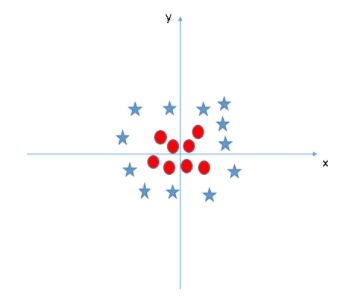SVM can solve this problem. Easily! It solves this problem by introducing an additional feature. Here, we will add a new feature z=x^2+y^2. Now, let’s plot the data points on axis x and z: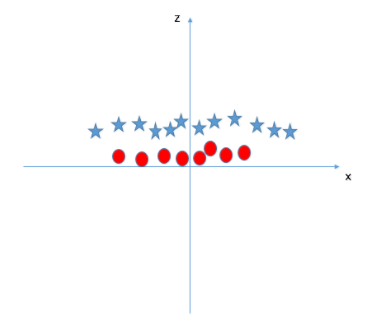In the above plot, points to consider are:

>> All values for z would be positive always because z is the squared sum of both x and y.
>> In the original plot, red circles appear close to the origin of x and y axes, leading to lower value of z and star relatively away from the original result to the higher value of z.

In SVM, it is easy to have a linear hyper-plane between these two classes. But, another burning question which arises is, should we need to add this feature manually to have a hyper-plane. No, SVM has a technique called the kernel trick. These are functions which take low dimensional input space and transform it to a higher dimensional space i.e. it converts not separable problem to separable problem, these functions are called kernels. It is mostly useful in non-linear separation problem. Simply put, it does some extremely complex data transformations, then find out the process to separate the data based on the labels or outputs you’ve defined.

When we look at the hyper-plane in the original input space it looks like a circle:

Related Article: Machine Learning Applications

### How to Compute Optimal Hyperplane

Let’s introduce the notation used to define formally a hyperplane:

where is known as the weight vector and as the bias.

The optimal hyperplane can be represented in an infinite number of different ways by scaling and. As a matter of convention, among all the possible representations of the hyperplane, the one chosen is

where symbolizes the training examples closest to the hyperplane. In general, the training examples that are closest to the hyperplane are called support vectors. This representation is known as the canonical hyperplane.

Now, we use the result of geometry that gives the distance between a point and a hyperplane:In particular, for the canonical hyperplane, the numerator is equal to one and the distance to the support vectors isRecall that the margin introduced in the previous section, here denoted as, is twice the distance to the closest examples:Finally, the problem of maximizingis equivalent to the problem of minimizing a functionsubject to some constraints. The constraints model the requirement for the hyperplane to classify correctly all the training examples. Formally,

whererepresents each of the labels of the training examples.>> high-dimensionality - The SVM is an effective tool in high-dimensional spaces, which is particularly applicable to document classification and sentiment analysis where the dimensionality can be extremely large (≥106).
>> Memory Efficiency - Since only a subset of the training points is used in the actual decision process of assigning new members, only these points need to be stored in memory (and calculated upon) when making decisions.
>> Versatility - Class separation is often highly non-linear. The ability to apply new kernels allows substantial flexibility for the decision boundaries, leading to greater classification performance.

>> `p>n- `In situations where the number of features for each object (p) exceeds the number of training data samples (n), SVMs can perform poorly. This can be seen intuitively as if the high-dimensional feature space is much larger than the samples, then there are less effective support vectors on which to support the optimal linear hyperplanes, leading to poorer classification performance as new unseen samples are added.
>> Non-Probabilistic - Since the classifier works by placing objects above and below a classifying hyperplane, there is no direct probabilistic interpretation for group membership. However, one potential metric to determine "effectiveness" of the classification is how far from the decision boundary the new point is.

Frequently Asked Machine Learning Interview Questions

#### Python Example

The most widely used library for implementing machine learning algorithms in Python is scikit-learn. The class used for SVM classification in scikit-learn is  `svm.SVC()`

`sklearn.svm.SVC (C=1.0, kernel='rbf', degree=3, gamma='auto')`

#### Parameters are as follows:

1. C: It is the regularization parameter, C, of the error term.
2. kernel: It specifies the kernel type to be used in the algorithm. It can be ‘linear’, ‘poly’, ‘rbf’, ‘sigmoid’, ‘precomputed’, or a callable. The default value is ‘rbf'.
3. degree: It is the degree of the polynomial kernel function (‘poly’) and is ignored by all other kernels. The default value is 3.
4. gamma: It is the kernel coefficient for ‘rbf’, ‘poly’, and ‘sigmoid’. If gamma is ‘auto’, then 1/n_features will be used instead.

``````from sklearn import datasets, svm
from sklearn.model_selection import train_test_split
from sklearn.metrics import confusion_matrix
X = iris.data[:, :2]
y = iris.target
#Split the data into test and train
X_train, X_test, y_train, y_test = train_test_split(X, y, test_size=0.3, random_state=0)

# Linear Kernel
svc_linear = svm.SVC(kernel='linear', C=1)
svc_linear.fit(X_train, y_train)
predicted= svc_linear.predict(X_test)
cnf_matrix = confusion_matrix(y_test, predicted)
print(cnf_matrix)
output

[[16  0  0]

[ 0 13  5]

[ 0 4 7]``````

This is the "Iris" dataset. Originally published at UCI Machine Learning Repository: Iris Data Set, this small dataset from 1936 is often used for testing out machine learning algorithms and visualizations (for example, Scatter Plot). Each row of the table represents an iris flower, including its species and dimensions of its botanical parts, sepal and petal, in centimetresCourse Schedule
NameDates
Machine Learning TrainingJun 03 to Jun 18
Machine Learning TrainingJun 06 to Jun 21
Machine Learning TrainingJun 10 to Jun 25
Machine Learning TrainingJun 13 to Jun 28
Last updated: 31 May 2023